##### The Japanese Bonsai specialist
Direct order Contact Help / Services Newsletter# Kokufu-ten bonsai exhibition catalogue 85 (2011)

kokufu ten catalogue de l'exposition 2012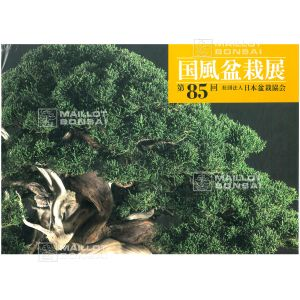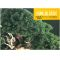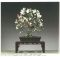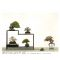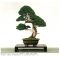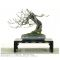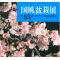ref. : 3220

95,00

This item is temporarily unavailableNotify availability

###### Description

Imported from Japan

Language: Japanese

Author: Japan Bonsai Association

Dimensions: 26 x 25.2 x 2.4 cm

280 pages in full colour

The highly collectible bonsai catalogue No.85 from the famous Kokufu-ten, an annual Japanese national bonsai show dating back to 1934. The event, held in Tokyo, is one of the biggest bonsai exhibitions in the world. The 2012 book features colour photos of the most beautiful masterpieces, including unique specimens, large trees, miniature bonsai, ancient pots and furniture. Peruse the work of Japan's premier bonsai artists in this picture-led edition.

#bonsai 8.6 #catalogue 3.9 #kokufu 3.6 #japan 3.5 #exhibition 3 #japanese 2.8 #colour 2.6 #books 2.5 #from 2.4 #masterpieces 2.2

Formule
(( ROUND((CHAR_LENGTH(b.article_nom)-CHAR_LENGTH(REPLACE(b.article_nom, 'bonsai', '')))/LENGTH('bonsai')) + ROUND((CHAR_LENGTH(b.article_description)-CHAR_LENGTH(REPLACE(b.article_description, 'bonsai', '')))/LENGTH('bonsai')) ) * 8.6) + (( ROUND((CHAR_LENGTH(b.article_nom)-CHAR_LENGTH(REPLACE(b.article_nom, 'catalogue', '')))/LENGTH('catalogue')) + ROUND((CHAR_LENGTH(b.article_description)-CHAR_LENGTH(REPLACE(b.article_description, 'catalogue', '')))/LENGTH('catalogue')) ) * 3.9) + (( ROUND((CHAR_LENGTH(b.article_nom)-CHAR_LENGTH(REPLACE(b.article_nom, 'kokufu', '')))/LENGTH('kokufu')) + ROUND((CHAR_LENGTH(b.article_description)-CHAR_LENGTH(REPLACE(b.article_description, 'kokufu', '')))/LENGTH('kokufu')) ) * 3.6) + (( ROUND((CHAR_LENGTH(b.article_nom)-CHAR_LENGTH(REPLACE(b.article_nom, 'japan', '')))/LENGTH('japan')) + ROUND((CHAR_LENGTH(b.article_description)-CHAR_LENGTH(REPLACE(b.article_description, 'japan', '')))/LENGTH('japan')) ) * 3.5) + (( ROUND((CHAR_LENGTH(b.article_nom)-CHAR_LENGTH(REPLACE(b.article_nom, 'exhibition', '')))/LENGTH('exhibition')) + ROUND((CHAR_LENGTH(b.article_description)-CHAR_LENGTH(REPLACE(b.article_description, 'exhibition', '')))/LENGTH('exhibition')) ) * 3) + (( ROUND((CHAR_LENGTH(b.article_nom)-CHAR_LENGTH(REPLACE(b.article_nom, 'japanese', '')))/LENGTH('japanese')) + ROUND((CHAR_LENGTH(b.article_description)-CHAR_LENGTH(REPLACE(b.article_description, 'japanese', '')))/LENGTH('japanese')) ) * 2.8) + (( ROUND((CHAR_LENGTH(b.article_nom)-CHAR_LENGTH(REPLACE(b.article_nom, 'colour', '')))/LENGTH('colour')) + ROUND((CHAR_LENGTH(b.article_description)-CHAR_LENGTH(REPLACE(b.article_description, 'colour', '')))/LENGTH('colour')) ) * 2.6) + (( ROUND((CHAR_LENGTH(b.article_nom)-CHAR_LENGTH(REPLACE(b.article_nom, 'from', '')))/LENGTH('from')) + ROUND((CHAR_LENGTH(b.article_description)-CHAR_LENGTH(REPLACE(b.article_description, 'from', '')))/LENGTH('from')) ) * 2.4) + (( ROUND((CHAR_LENGTH(b.article_nom)-CHAR_LENGTH(REPLACE(b.article_nom, 'masterpieces', '')))/LENGTH('masterpieces')) + ROUND((CHAR_LENGTH(b.article_description)-CHAR_LENGTH(REPLACE(b.article_description, 'masterpieces', '')))/LENGTH('masterpieces')) ) * 2.2) + (( ROUND((CHAR_LENGTH(b.article_nom)-CHAR_LENGTH(REPLACE(b.article_nom, 'association', '')))/LENGTH('association')) + ROUND((CHAR_LENGTH(b.article_description)-CHAR_LENGTH(REPLACE(b.article_description, 'association', '')))/LENGTH('association')) ) * 2.1)

## Secure payment## Delivery

Our logistic partners :